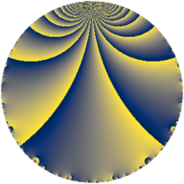# Properties

 Label 8712.2.a.uLevel $8712$ Weight $2$ Character orbit 8712.a Self dual yes Analytic conductor $69.566$ Analytic rank $0$ Dimension $1$ CM no Inner twists $1$

# Related objects

## Newspace parameters

 Level: $$N$$ $$=$$ $$8712 = 2^{3} \cdot 3^{2} \cdot 11^{2}$$ Weight: $$k$$ $$=$$ $$2$$ Character orbit: $$[\chi]$$ $$=$$ 8712.a (trivial)

## Newform invariants

 Self dual: yes Analytic conductor: $$69.5656702409$$ Analytic rank: $$0$$ Dimension: $$1$$ Coefficient field: $$\mathbb{Q}$$ Coefficient ring: $$\mathbb{Z}$$ Coefficient ring index: $$1$$ Twist minimal: no (minimal twist has level 24) Fricke sign: $$-1$$ Sato-Tate group: $\mathrm{SU}(2)$

## $q$-expansion

 $$f(q)$$ $$=$$ $$q + 2q^{5} + O(q^{10})$$ $$q + 2q^{5} + 2q^{13} + 2q^{17} + 4q^{19} + 8q^{23} - q^{25} + 6q^{29} + 8q^{31} + 6q^{37} - 6q^{41} - 4q^{43} - 7q^{49} + 2q^{53} - 4q^{59} + 2q^{61} + 4q^{65} - 4q^{67} - 8q^{71} - 10q^{73} + 8q^{79} - 4q^{83} + 4q^{85} + 6q^{89} + 8q^{95} + 2q^{97} + O(q^{100})$$

## Embeddings

For each embedding $$\iota_m$$ of the coefficient field, the values $$\iota_m(a_n)$$ are shown below.

For more information on an embedded modular form you can click on its label.

Label $$\iota_m(\nu)$$ $$a_{2}$$ $$a_{3}$$ $$a_{4}$$ $$a_{5}$$ $$a_{6}$$ $$a_{7}$$ $$a_{8}$$ $$a_{9}$$ $$a_{10}$$
1.1
 0
0 0 0 2.00000 0 0 0 0 0
 $$n$$: e.g. 2-40 or 990-1000 Significant digits: Format: Complex embeddings Normalized embeddings Satake parameters Satake angles

## Atkin-Lehner signs

$$p$$ Sign
$$2$$ $$-1$$
$$3$$ $$-1$$
$$11$$ $$-1$$

## Inner twists

This newform does not admit any (nontrivial) inner twists.

## Twists

By twisting character orbit
Char Parity Ord Mult Type Twist Min Dim
1.a even 1 1 trivial 8712.2.a.u 1
3.b odd 2 1 2904.2.a.c 1
11.b odd 2 1 72.2.a.a 1
12.b even 2 1 5808.2.a.s 1
33.d even 2 1 24.2.a.a 1
44.c even 2 1 144.2.a.b 1
55.d odd 2 1 1800.2.a.m 1
55.e even 4 2 1800.2.f.c 2
77.b even 2 1 3528.2.a.d 1
77.h odd 6 2 3528.2.s.j 2
77.i even 6 2 3528.2.s.y 2
88.b odd 2 1 576.2.a.d 1
88.g even 2 1 576.2.a.b 1
99.g even 6 2 648.2.i.g 2
99.h odd 6 2 648.2.i.b 2
132.d odd 2 1 48.2.a.a 1
165.d even 2 1 600.2.a.h 1
165.l odd 4 2 600.2.f.e 2
176.i even 4 2 2304.2.d.k 2
176.l odd 4 2 2304.2.d.i 2
220.g even 2 1 3600.2.a.v 1
220.i odd 4 2 3600.2.f.r 2
231.h odd 2 1 1176.2.a.i 1
231.k odd 6 2 1176.2.q.a 2
231.l even 6 2 1176.2.q.i 2
264.m even 2 1 192.2.a.d 1
264.p odd 2 1 192.2.a.b 1
308.g odd 2 1 7056.2.a.q 1
396.k even 6 2 1296.2.i.e 2
396.o odd 6 2 1296.2.i.m 2
429.e even 2 1 4056.2.a.i 1
429.l odd 4 2 4056.2.c.e 2
528.s odd 4 2 768.2.d.d 2
528.x even 4 2 768.2.d.e 2
561.h even 2 1 6936.2.a.p 1
627.b odd 2 1 8664.2.a.j 1
660.g odd 2 1 1200.2.a.d 1
660.q even 4 2 1200.2.f.b 2
924.n even 2 1 2352.2.a.i 1
924.y even 6 2 2352.2.q.r 2
924.z odd 6 2 2352.2.q.l 2
1320.b odd 2 1 4800.2.a.cc 1
1320.u even 2 1 4800.2.a.q 1
1320.bn odd 4 2 4800.2.f.d 2
1320.bt even 4 2 4800.2.f.bg 2
1716.m odd 2 1 8112.2.a.be 1
1848.b odd 2 1 9408.2.a.h 1
1848.e even 2 1 9408.2.a.cc 1

By twisted newform orbit
Twist Min Dim Char Parity Ord Mult Type
24.2.a.a 1 33.d even 2 1
48.2.a.a 1 132.d odd 2 1
72.2.a.a 1 11.b odd 2 1
144.2.a.b 1 44.c even 2 1
192.2.a.b 1 264.p odd 2 1
192.2.a.d 1 264.m even 2 1
576.2.a.b 1 88.g even 2 1
576.2.a.d 1 88.b odd 2 1
600.2.a.h 1 165.d even 2 1
600.2.f.e 2 165.l odd 4 2
648.2.i.b 2 99.h odd 6 2
648.2.i.g 2 99.g even 6 2
768.2.d.d 2 528.s odd 4 2
768.2.d.e 2 528.x even 4 2
1176.2.a.i 1 231.h odd 2 1
1176.2.q.a 2 231.k odd 6 2
1176.2.q.i 2 231.l even 6 2
1200.2.a.d 1 660.g odd 2 1
1200.2.f.b 2 660.q even 4 2
1296.2.i.e 2 396.k even 6 2
1296.2.i.m 2 396.o odd 6 2
1800.2.a.m 1 55.d odd 2 1
1800.2.f.c 2 55.e even 4 2
2304.2.d.i 2 176.l odd 4 2
2304.2.d.k 2 176.i even 4 2
2352.2.a.i 1 924.n even 2 1
2352.2.q.l 2 924.z odd 6 2
2352.2.q.r 2 924.y even 6 2
2904.2.a.c 1 3.b odd 2 1
3528.2.a.d 1 77.b even 2 1
3528.2.s.j 2 77.h odd 6 2
3528.2.s.y 2 77.i even 6 2
3600.2.a.v 1 220.g even 2 1
3600.2.f.r 2 220.i odd 4 2
4056.2.a.i 1 429.e even 2 1
4056.2.c.e 2 429.l odd 4 2
4800.2.a.q 1 1320.u even 2 1
4800.2.a.cc 1 1320.b odd 2 1
4800.2.f.d 2 1320.bn odd 4 2
4800.2.f.bg 2 1320.bt even 4 2
5808.2.a.s 1 12.b even 2 1
6936.2.a.p 1 561.h even 2 1
7056.2.a.q 1 308.g odd 2 1
8112.2.a.be 1 1716.m odd 2 1
8664.2.a.j 1 627.b odd 2 1
8712.2.a.u 1 1.a even 1 1 trivial
9408.2.a.h 1 1848.b odd 2 1
9408.2.a.cc 1 1848.e even 2 1

## Hecke kernels

This newform subspace can be constructed as the intersection of the kernels of the following linear operators acting on $$S_{2}^{\mathrm{new}}(\Gamma_0(8712))$$:

 $$T_{5} - 2$$ $$T_{7}$$ $$T_{13} - 2$$ $$T_{17} - 2$$

## Hecke characteristic polynomials

$p$ $F_p(T)$
$2$ $$T$$
$3$ $$T$$
$5$ $$-2 + T$$
$7$ $$T$$
$11$ $$T$$
$13$ $$-2 + T$$
$17$ $$-2 + T$$
$19$ $$-4 + T$$
$23$ $$-8 + T$$
$29$ $$-6 + T$$
$31$ $$-8 + T$$
$37$ $$-6 + T$$
$41$ $$6 + T$$
$43$ $$4 + T$$
$47$ $$T$$
$53$ $$-2 + T$$
$59$ $$4 + T$$
$61$ $$-2 + T$$
$67$ $$4 + T$$
$71$ $$8 + T$$
$73$ $$10 + T$$
$79$ $$-8 + T$$
$83$ $$4 + T$$
$89$ $$-6 + T$$
$97$ $$-2 + T$$